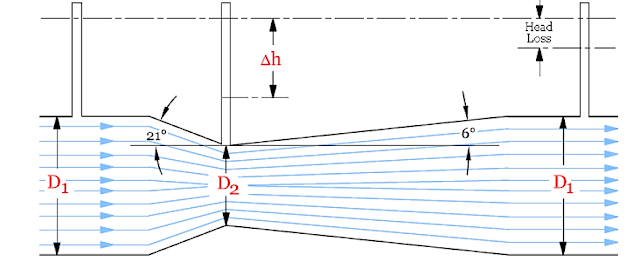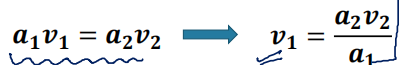# Part #4

## Fluid Dynamics

➢ Dynamics of fluid flow describes the fluid flow and its consequences with consideration of the nature of forces causing the motion, and effect of various forces on the flow.
➢ In this energy of fluid is also considered.

## Equations of Motion for Fluid Flow

➢ According to Newton’s second law of motion, the net force Fx acting on a fluid element in the direction of x is equal to mass m of the fluid element multiplied by the acceleration ax in the x-direction.

➢ Therefore we have to find net force acting on the fluid element, for that we have to describe all the forces acting on the fluid element.

### Equations of Motion for Fluid Flow

Forces on a Fluid Element in A Flow:
➢ Fg gravity force
➢ Fp the pressure force
➢ Fv Viscous force
➢ Ft force due to turbulence
➢ Fc force due to compressibility
➢ Thus in equation, the net force will be :
F = Fg + Fp + Fv + Ft + Fc
➢ If the force due to compressibility is negligible(incompressible flow), the resulting net force
F = Fg + Fp + Fv + Ft + Fc
➢ And equations of motion are called Reynold’s equation of motion.
➢ If the force due to turbulence is negligible (laminar flow), the resulting net force
F = Fg + Fp + Fv
➢ And equations of motion are called Navier-Stokes Equation of motion.
➢ If the Viscous force is negligible (Ideal flow), the resulting net force
F = Fg + Fp
➢ And equations of motion are called Euler’s equation of motion.

## EULER’S EQUATION OF MOTION

➢ This is equation of motion in which the forces due to gravity and pressure are taken into consideration.
➢ This is derived by considering the motion of a fluid element along a steam line.
➢ Consider a stream line in which flow is taking place in s- direction as shown in figure.
➢ Consider a cylindrical element of cross section dA and length ds.
➢ The forces acting on the cylindrical elementare:
➢ Pressure force pdA in the direction of flow.
➢ Pressure force (p + dp/ds.ds)dA opposite to the direction of flow
➢ Weight of element ρg dA ds.

Let θ is the angle between the direction of flow and the line of action of the weight of element.
➢ By newtons law in s-direction :
➢ By putting value in the equation we get:
➢ By putting value of in the equation we get:  ➢ This equation is known as Euler's equation of motion.

## BERNOULLI’S EQUATION

➢ Bernoulli’s equation is obtained by integrating the Euler’sequation of motion.
➢ This equation is basically a form of energy conservation law.
➢ The following are the assumptions made in the derivation of Bernoulli’s equation:
(i) The fluid is ideal, i.e. viscosity is zero
(iii) The flow is incompressible
(iv) The flow is irrotational
➢ By integrating the Euler’s equation of motion we get :

➢ P/ρg = pressure energy per unit weight of fluid or pressure head.
➢ V2 / 2g = kinetic energy per unit weight or kinetic head.
➢ Z = potential energy per unit weight or potential head.

## Different forms of Bernoulli's equation :

In the form of energy per unit mass : P/ρ+ gz + v2/2 =constant
In the form of energy per unit Weight : P/ρg + v2/2g + z = constant
In the form of energy per unit Volume: P + ρv2/2 +ρgz = constant

## BERNOULLI’S EQUATION FOR REAL FLUID

➢ The Bernoulli’s equation was derived on the assumption that fluid is inviscid (non-viscous) and therefore frictionless. But all the real fluids are viscous and hence offer resistance to flow.
➢ Thus there are always some losses in fluid flows and hence in the application of Bernoulli’s equation, these losses have to be taken into consideration. Thus the Bernoulli’s equation for real fluids between point 1 and 2 is given as

➢ Where hL is loss of energy between points 1 and 2.
➢ In case of rotational flow Bernoulli’s equation is not valid at every point, but it can be applied on a single stream line in rotational flow.

## PRACTICAL APPLICATIONS OF BERNOULLI’S EQUATION

➢ Bernoulli’s equation is applied in all problems of incompressible fluid flow where energy considerations are involved. But we shall consider its application to the following measuring devices:
(i) Venturi meter.
(ii) Orifice meter.
(iii) Pitot-tube.

## Venturi-Meter

➢ A venturi-meter is a device used for measuring the rate of a flow of a fluid flowing through a pipe.
➢ It is based on the Principle of Bernoulli’s equation.
➢ It consists of three parts:
(i) A short converging part
(ii) Throat,
(iii) Diverging part.

## Design Parameter of Venturi-Meter

### Converging part➢ It is starting section of venturi-meter which attached at inlet pipe. The cross sectional area of this cone starts to decrease and the converging angle is 21 degree.
➢ Its length is 2.7*(D1 - D2).
➢ Here (D1) is the diameter of inlet section and (D2) is the diameter of throat. Other end of converging is attached with throat.

### Throat

➢ Throat is middle portion of venturi-meter and its cross sectional area is too small. At this point pressure is decreases and velocity is increases.
➢ One end is connected with converging part and other end is attached with diverging part.
➢ Diameter of throat is ¼ to ¾ of the diameter of the inlet pipe, but mostly it is ½ of the diameter of the pipe.

### Diverging part

➢ Diverging part is last part of venturi-meter and its cross sectional area is increases continually. Angle of diverging part is 5 to 12 degree. Its cross sectional area continuously increases. One end
is connected to throat and other end is connected to outlet pipe .
➢ The main reason behind the low diverging angle is to avoid the formation of eddies because flow separation and eddies formation will results in large amount of loss in energy

### Expression for rate of flow through Venturi-Meter

➢ Let d₁ = diameter at inlet or at section 1
➢ P₁ = pressure at section 1
➢ v₁ = velocity of fluid at section 1
➢ a1 = area at section = π/4 d21 and d₂, P₂, v₂, a₂ are corresponding values at section 2

➢ Applying Bernoulli’s equation at section 1 and 2 we get :➢ By putting the value in the equation :➢ Equation gives the discharge under ideal conditions and is called, theoretical discharge. Actual discharge will be less than theoretical discharge.
➢ Where Cd = Co-efficient of venturi-meter and its value is less than Generally 0.98 to 0.99.
Value of ‘h’ Given By Differential U-Tube Manometer
➢ Let
➢ Sh = Sp. gravity of the heavier liquid in manometer
➢ So = Sp. Gravity of the liquid flowing through pipe
➢ X = Difference of the heavier liquid column in U-tube

## QUESTIONS FROM THESE TOPICS:

Which one of the following assumptions of Bernoulli’s theorem is not correct?
(A) Flow should not be unsteady
(B) Flow should be continuous
(C) The fluid should be compressible
(D) Flow should be frictionless

Each term of Bernoulli’s equations stated in form
p/w + v2/2g + y = constant has unit of
(A) N
(B) Nm/kg
(C) m
(D) Nm/s

A liquid flows with constant velocity from low level Z1, pressure P1 to a higher level Z2, pressure P2. It can be concluded
(A) First law of thermodynamics has been violated
(B) Second law of thermodynamics has been violated
(C) Z2 < Z1
(D) P2 < P1
The fluid forces considered in the Navier Stokes equation are ____ .
(A) Gravity, pressure and viscous
(B) Gravity, pressure and turbulent
(C) Pressure, viscous and turbulent
(D) Gravity, viscous and turbulent

Euler’s equation for the motion of liquid assumes that _____.
(A) Fluid is viscous
(B) Fluid is homogeneous and incompressible
(C) Velocity of flow is non uniform over the section
(D) Flow is unsteady along with stream line

The total energy of each particle at various places in the case of perfect incompressible fluid flowing in continuous stream
(A) Keeps on increasing
(B) Keeps on decreasing
(C) Remains constant
(D) May increase or decrease

Bernoulli’s equation cannot be applied when the flow is ____ .
(A) Rotational
(B) Turbulent
(D) All options are correct

Bernoulli equation deals with the law of conservation of
(A) Mass
(B) Momentum
(C) Energy
(D) Work

Bernoulli's equation represents the
(a) Forces at any point in the flow field and is obtained by integrating the momentum equation for viscous flows.
(b) Energies at any point in the flow field and is obtained by integrating the Euler equations.
(c) Momentum at any point in the flow field and is obtained by integrating the equation of continuity.
(d) Moment of momentum and is obtained by integrating the energy equation.

THANK YOU VISIT AND SHARE..